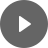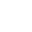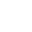8.3 Hyperbola - StudyNinja
Select Page

HSC Standard Maths Resources

Browse: 1. Home  »  8. Non-linear Relationship »  8.3 Hyperbola

8.3 Hyperbola

A hyperbola curve is formed when there is reciprocal relationship between dependent and independent variables.Example 1

Draw the graph of reciprocal equation y = 4/x – 8. What are the asymptotes? Is there any x-intercept?

Example 2

Which one of the following equations best describes the graph below?Get access to 20 Mock Exams with over 700 exam-style questions for HSC Standard Maths.

You might find the following videos helpful related to this section:8E Reciprocal Functions (1 of 3)

by Mr Bodgers (click to view channel)

Introduces the reciprocal function and shows what a hyperbolic curve looks like.8E Reciprocal Functions (2 of 3)

by Mr Bodgers (click to view channel)

Shows you how to perform transformation on basic hyperbolas using Desmos.8E Reciprocal Functions (3 of 3)

by Mr Bodgers (click to view channel)

This is an interactive graphing activity. You are required to construct hyperbolas in Desmos that meet certain criteria.8F Reciprocal Models

by Mr Bodgers (click to view channel)

Models a practical problem using a reciprocal function. In this example we use a reciprocal equation to calculate the cost per person for hiring a bus.8G Inverse Variation (1 of 2)

by Mr Bodgers (click to view channel)

Explains what inverse variation is and completes an example.8G Inverse Variation (2 of 2)

by Mr Bodgers (click to view channel)

Completes a practical problem on inverse variation.The following are the types of questions you can expect in exam:

Study notes of this section and other resources can be accessed here:

Browse: 1. Home  »  8. Non-linear Relationship »  8.3 Hyperbola